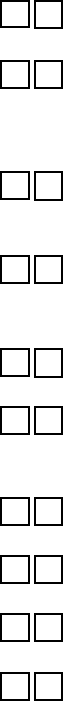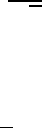Study Guides (400,000)
US (230,000)
UCLA (3,000)
MATH (300)
All (20)
Final

# MATH 33AH Lecture Notes - Lecture 6: Orthogonal Matrix, Invertible Matrix, BecquerelExam

Department
Mathematics
Course Code
MATH 33AH
Professor
All
Study Guide
Final

This preview shows pages 1-2. to view the full 8 pages of the document.
MATH 33A LECTURE 3
2ND MIDTERM SOLUTIONS
FALL 2016
2016 UC Regents/Dimitri Shlyakhtenko.
Copying or unauthorized distribution or transmission by any means expressly prohibited.

Only pages 1-2 are available for preview. Some parts have been intentionally blurred.MATH 33A LECTURE 3 2ND MIDTERM SOLUTIONS FALL 2016 2
Problem 1. (True/False, 1 pt each) Mark your answers by ﬁlling in the appropriate box
next to each question.
(i: T F ) Any basis for R3consists of 3 orthonormal vectors. (False): e.g.
1
0
0
,
1
1
0
,
1
1
1
(ii: T F ) If Ais a 4 ×4 orthogonal matrix, Ae1=e2,Ae2=e3,Ae3=e1, then Ae4=e4or
Ae4=e4(True): if w=Ae4then wmust be perpendicular to the other columns
of A, i.e., to e2,e3and e1so be a multiple of e4. But since its length must be 1,
w=±e4
(iii: T F ) There are no vectors v,win R7so that v·w=7 and kvk=kwk=2. (True):
The Cauchy-Schwartz inequality states that |vw| ≤ kvkkwkwhich would mean
72·2 which cannot be true.
(iv: T F )(Atx)y=x(Ay)for any vectors x,yand any matrix A(here denotes the
dot product) (True): if Ahas entries aij,xhas entries xiand yhas entries yithen
Atxy=ij yjaij xi=ij aij xiyjwhile x(Ay) = ij xiaijyj=ij xiyjaij.
(v: T F ) A square orthogonal matrix is invertible. (True) Since AtA=I,Acannot have any
kernel, so by rank nullity has full rank, so is invertible.
(vi: T F ) If AtA=Ithen Ais an orthogonal matrix. (True) if Ahas columns v1, . . . , vnthen
the i,j-th entry of AtAis exactly vivj; so Ais orthogonal iff vivjis zero for i6=j
and 0 if i=ji.e., AtA=I.
(vii: T F ) Every basis for the plane x+y+z=0 consists of two vectors. (True) the dimen-
sion of the plane is 2.
(viii: T F ) The columns of an orthogonal matrix are orthonormal. (True) this is the deﬁnition
of an orthogonal matrix.
(ix: T F ) The determinant of an invertible matrix is nonzero. (True) 1 =det I=det AA1=
(det A)·(det A1)so det A6=0.
(x: T F ) For any matrix A, the kernel of Ais perpendicular to the image of At. (True) see
book.

Unlock to view full version

Only pages 1-2 are available for preview. Some parts have been intentionally blurred.MATH 33A LECTURE 3 2ND MIDTERM SOLUTIONS FALL 2016 3
Problem 2. (10 pts) Let Pbe the subspace of R4consisting of vectors
x
y
z
w
satisfying
x+y=wand x+z=w.
(a) Find a basis for P.
Note that by deﬁnition Pconsists of those vectors which satisfy
x+y+w=0, x+z+w=0
which means that
P=ker A
with
A=1101
1011.
Row-reducing
A1 1 0 1
01101 1 0 1
0 1 1 0 1 0 1 1
0 1 1 0
The last two columns correspond to free variables so that we have:
x
y
z
w
=a
1
1
1
0
+b
1
0
0
1
so that
1
1
1
0
and
1
0
0
1
form a basis. Note: other answers are possible; the two
vectors must lie in Pand be linearly independent.
(b) Find the dimension of P.
Since there are two basis vectors, the dimensino is 2.
(c) Find an orthonormal basis for P.
Using Gram-Schmidt we get:
w1=
1
1
1
0
,u1=1
3
1
1
1
0
w2=v2(v2u1)u1=
1
0
0
1
1
3
1
1
1
0
=
2/3
1/3
1/3
1